# Lawson Criterion

Consider a thermonuclear plasma that consists of an optimal 50%-50% mixture of deuterium and tritium ions, as well as electrons. Suppose that there are no impurity ions or helium ash particles (i.e., thermalized alpha particles) present in the plasma. Quasi-neutrality  demands that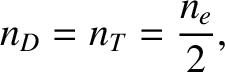(1.10)

where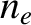is the number density of electrons. Suppose that the two ions species have the same temperature,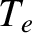(measured in energy units), as the electrons. The total thermal energy density of the plasma is thus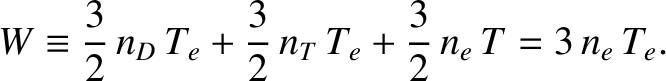(1.11)

The rate of nuclear fusion reactions per unit volume is [see Equation (1.6)]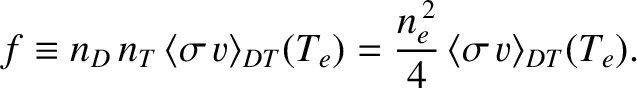(1.12)

In order to achieve a self-sustaining nuclear fusion reaction in a thermonuclear plasma, the fusion heating power per unit volume,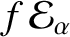, must exceed the energy loss rate per unit volume,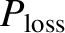. (Recall that the alpha particles produced by nuclear fusion reactions heat the plasma, whereas the neutrons exit the plasma without heating it.) Thus, we require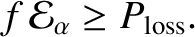(1.13)

Let us write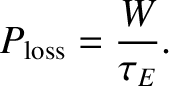(1.14)

Here, the energy confinement time,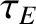, is a measure of how long the plasma's thermal energy is confined within the plasma before escaping. Note that, at this stage, we are making no statement about the nature of the energy loss mechanism. In fact, Equation (1.14) can be thought of as the definition of. The previous four equations can be combined to give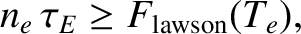(1.15)

where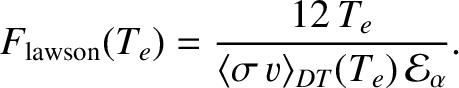(1.16)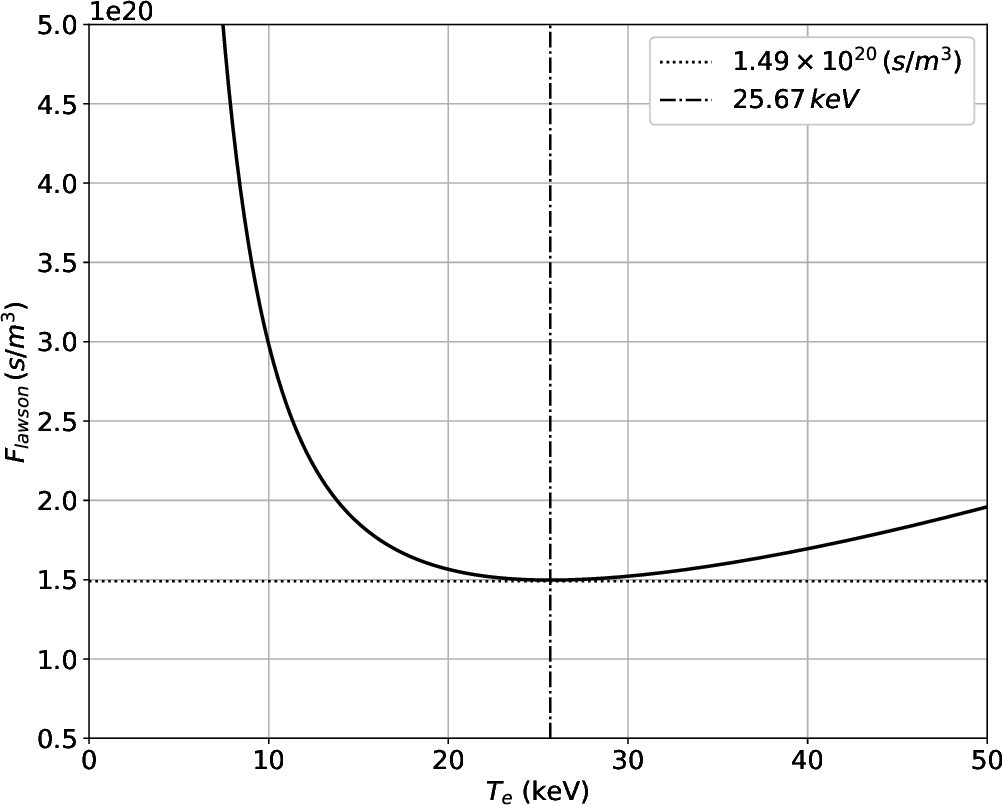Figure 1.2 plots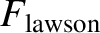as a function of the electron temperature,. It can be be seen that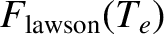attains a minimum value of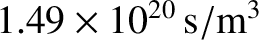when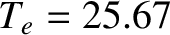keV. Thus, we conclude that a self-sustaining nuclear fusion reaction is only possible in a thermonuclear plasma if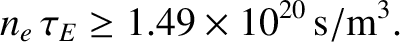(1.17)

This criterion is known as the Lawson criterion .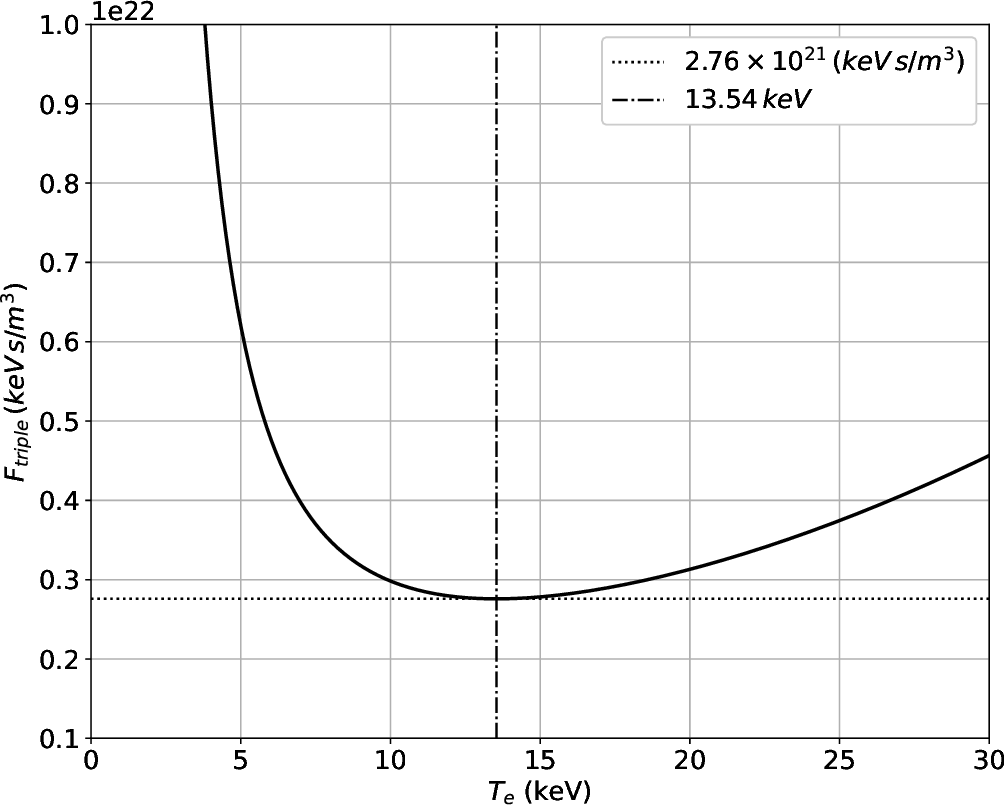In conventional magnetic confinement devices,andcan be varied over a wide range of values. However, the maximum value of the plasma pressure, which is proportional to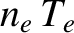, is fixed by plasma stability considerations [26,64]. (See Section 1.10.) Now, according to Equations (1.15) and (1.16), the criterion for a self-sustaining nuclear fusion reaction can be recast in the form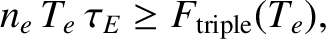(1.18)

where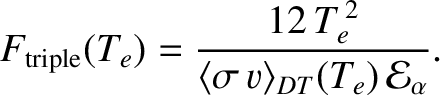(1.19)

Given that the maximum value of the productis fixed, it follows that fusion reactivity is maximized at the temperature that minimizes the function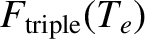. As illustrated in Figure 1.3,attains a minimum value of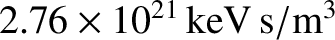when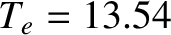keV. Thus, a more useful form of the Lawson criterion is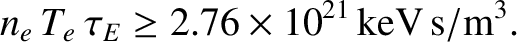(1.20)

Here,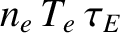is known as the fusion triple product, and is the conventional figure of merit for thermonuclear fusion reactions in magnetic confinement devices .